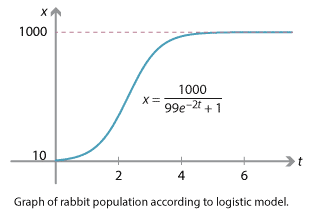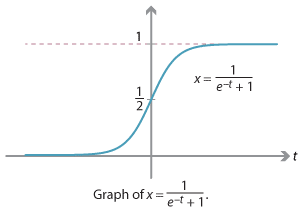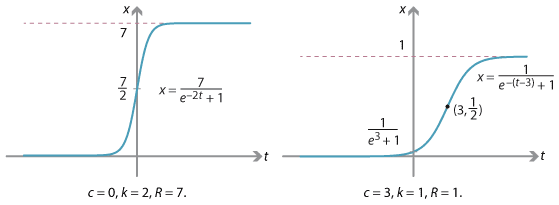### Logistic growth

We have seen many examples of exponential population growth based on an equation

$\dfrac{dx}{dt} = kx,$

saying intuitively that a larger population produces proportionately more offspring.

However, as we have discussed, exponential growth cannot continue forever in a finite ecosystem. There are always limits to growth. One way to model ecological constraints is by adding an extra factor corresponding to the carrying capacity $$R$$ of the ecosystem:

$\dfrac{dx}{dt} = kx \Bigl( 1 - \dfrac{x}{R} \Bigr).$

Here $$k$$ (the continuous growth rate) and $$R$$ are constants. This model is called a logistic model of growth. As $$x$$ increases from 0 to $$R$$, the factor $$1-\dfrac{x}{R}$$ decreases from 1 to 0, expressing the idea that as the population increases towards carrying capacity, growth becomes increasingly difficult; at carrying capacity, growth drops to zero.

Differential equations of this type can be solved explicitly, as we now illustrate.

Suppose there are initially 10 rabbits on an island with a carrying capacity of 1000. In the absence of ecological constraints, the rabbits reproduce with a growth rate of 2. Following the logistic model, we have

$\dfrac{dx}{dt} = 2 x \Bigl( 1 - \dfrac{x}{1000} \Bigr) = \dfrac{x (1000 - x)}{500}.$

Using the fact that $$\dfrac{dx}{dt} \cdot \dfrac{dt}{dx} = 1$$ gives

$\dfrac{dt}{dx} = \dfrac{500}{x(1000 - x)}.$

In order to integrate this expression we write it in terms of partial fractions, setting

$\dfrac{500}{x(1000 - x)} = \dfrac{A}{x} + \dfrac{B}{1000 - x}$

and solving for $$A$$ and $$B$$. Cross-multiplying gives $$500 = A(1000 - x) + Bx$$; so we obtain $$A = B = \dfrac{1}{2}$$. Thus we can write

$\dfrac{dt}{dx} = \dfrac{1}{2x} + \dfrac{1}{2(1000 - x)}.$

We may antidifferentiate term-by-term to obtain

\begin{align*} t &= \dfrac{1}{2} \log_e x - \dfrac{1}{2} \log_e (1000 - x) + c \\ &= \dfrac{1}{2} \log_e \Bigl(\dfrac{x}{1000-x}\Bigr) + c, \end{align*}

where $$c$$ is a constant. Rearranging this equation we obtain

$e^{2(t-c)} = \dfrac{x}{1000-x}.$

Substituting the initial condition $$t=0$$, $$x=10$$ gives $$e^{-2c} = \dfrac{1}{99}$$, so we have

$\dfrac{1}{99} e^{2t} = \dfrac{x}{1000 - x}.$

Solving now for $$x$$ gives

$x = \dfrac{1000}{99 e^{-2t} + 1}.$

This is the desired result. As $$t$$ increases towards infinity, $$e^{-2t}$$ approaches 0, so the denominator approaches 1, and therefore $$x$$ approaches the carrying capacity of 1000.Detailed description

Using the same technique, it can be shown that the general logistic model

$\dfrac{dx}{dt} = kx \Bigl( 1 - \dfrac{x}{R} \Bigr),$

with $$k, R$$ constants, has general solution

$x = \dfrac{R}{e^{-k(t-c)} + 1},$

where $$c$$ is a constant.

Exercise 10

Prove this.

Functions of this type are often called logistic functions. The simplest such function is

$f(t) = \dfrac{1}{e^{-t} + 1},$

which has the following graph.Detailed description

The next two graphs give further examples of logistic functions, with different values of $$k$$, $$R$$ and $$c$$.Detailed description

Next page - History and applications - World population